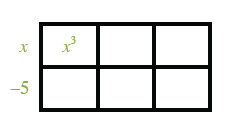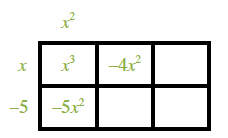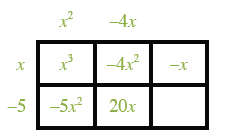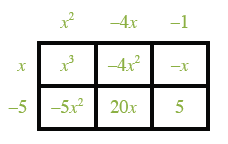Home > CCA2 > Chapter 8 > Lesson 8.3.1 > Problem8-124

8-124.

Now divide x3 − 9x2 + 19x + 5 by the factor that you chose in the preceding problem. If it is a factor, use it and the resulting factor to find all the zeros of the polynomial. If it is not a factor, reconsider your answer to the preceding problem and try a different factor. Homework Help ✎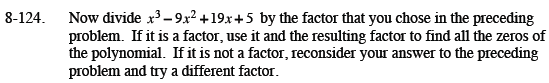x3 − 9x2 + 19x + 5 = (x − 5)(x2 − 4x − 1)

x − 5 = 0
x2 − 4x − 1 = 0

Now finish solving.